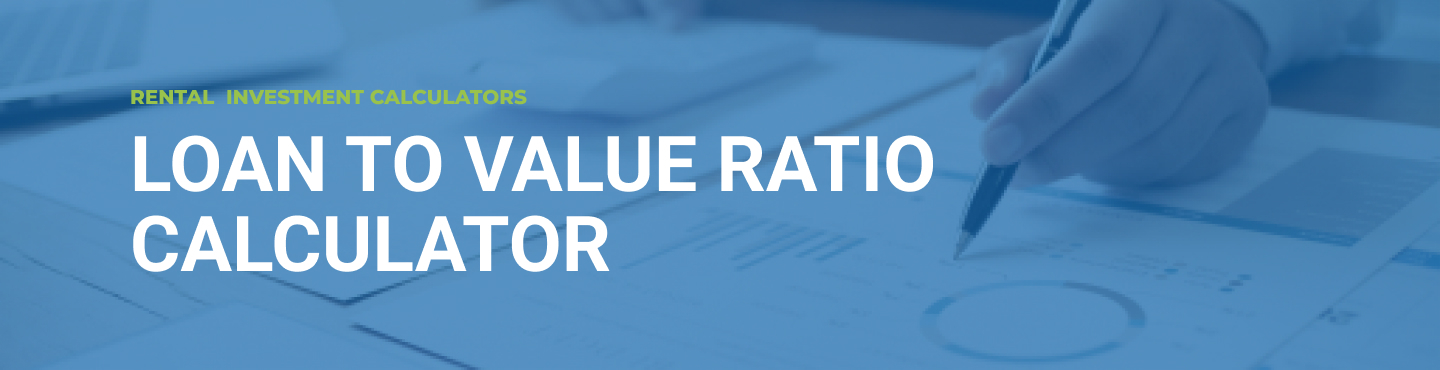# Loan to Value Ratio Calculator: How to Calculate LTV Ratio Easily

## What is loan to value ratio  (LTV)?

The loan to value ratio (LTV) is the calculation of credit risk that lenders and financial institutions study before approving a mortgage. Credit ratings with high LTV ratios are usually recognized as high risk loans.

If you decide to buy a property using a mortgage, you may need to calculate what part of your equity you have accumulated in your real estate investment. This indicator is used to determine the risk associated with a mortgage loan.

## How to calculate loan to value ratio?

The loan-to-value ratio can be calculated by knowing the total mortgage amount and the value of the home. The loan to value ratio formula  calculation is as follows:

LTV Ratio=Mortgage Amount/Home Value

You can use our loan to value ratio calculator to reduce the time for formula calculations.

## What is a good loan to value ratio?

The lowest LTV ratio is achieved due to lower real estate value and higher initial own contribution. Usually the LTV ratio is less than 80%. This value is good and means that the total mortgage is 80% of the total value of the house. The other 20% is paid from own funds and is part of the investor’s ownership of the house at the time of the mortgage. The lower the LTV ratio, the greater the investor’s share of his real estate, and the smaller the share of the loan.

## How to affect your LTV

Here are some factors to look out for if your  loan to value ratio is too high:

• Can you pay a higher down payment? By accumulating more savings or, say, inheriting, you will be able to make a larger down payment and probably get better mortgage terms.

• Choose a lower value property. When you buy a cheaper property, the initial down payment will also be lower, and therefore so would the LTV.

For example, suppose you save \$ 50,000 on your down payment. If the value of the property was \$ 500,000, this would result in a loan to value ratio of 90% (\$ 450,000 / \$ 500,000), whereas if the house price was \$ 300,000, the LTV ratio would be reduced to 83% (\$ 250,000 / \$ 300,000).

• Regularly repay the principal amount of the real estate loan. The decrease in the LTV ratio will occur gradually if you regularly pay monthly payments based on the payment schedule thus repaying your loan in full by the end of the term. By paying larger monthly payments, your principal amount of the loan will be repaid faster, and thus the LTV ratio will decrease.